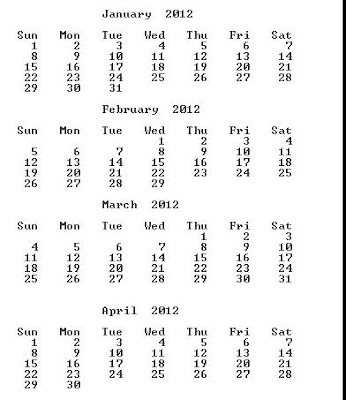# Java program to print Calendar of any given year in format

The below java program prints Calendar in format for any given year. Steps to be followed to print Calendar of any given year are as follows. The main requirement for the program is

1)  Calculation of day of week

2)  Leap year checking

3) Printing of days in format.

4) Initialize necessary arrays as given below

Now the number of days for each month is printed in format. Now we have to find where to start the day “1” to print of each month . For that we have to calculate the dow of the month,year and date 1. if dow is 5 means it is Friday, so we have to print the date 1 below Friday. So the number of spaces to be printed are calculated based on day of week. Then each day will be printed in a fixed space. String.format is used to print spaces in out program.

The complete java program is given below. It accepts the year  through command line.  To run the program, type In the command prompt

java calendar year

java calendar 2012

-prints the calendar for the year 2012

Sample out put of Calendar Year 2012